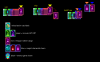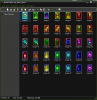# Legacy GMcard mechanic problem

#### phillipPbor

##### Member
I went to look for help and with out the help of discord I would not make something like this.

the cards are action cards...
giving player an opportunity to perform.
like kingdom hearts chains of memories.
has 3 buttons, I use 2.
there use, skip, and hold.

but I came up with problems, one of them is about the reload card...
after used up all the cards, when I press reload, all the cards came back leaving one from a start gone missing...and worse because I cant reach the reload button after used up attack magic cards.reload card is I thought was ment to be at the far end always but it proves I was wrong...
the reload card will be amongs a cards... the truth is that I did not make such mechanic alone...

deck_controller
create
Code:
``````usebutton = true;
/// the deck set up WIP
global.card_index = 0
global.use = 0
//card set.........................
current_cards = ds_list_create();   perma_cards = ds_list_create(); //v
//                     v                         |
tempa_used = ds_list_create();//  |
//                                    v          |
deck = ds_list_create();// <<<
//permacards
//tempacards
//battle start
for (var i = 0; i < ds_list_size(perma_cards); i++) {
}
for (var i = 0; i < ds_list_size(current_cards); i++) {
}
for (var i = 0; i < ds_list_size(tempa_used); i++) {
}
//setup.................&
num_of_cards = ds_list_size(deck);
xPos = 32
yPos = 184
for(var i = 0; i <=num_of_cards; i++)
{
//place cards
var curr_card = instance_create(xPos,yPos,card_obj);
if (i == 0)
{
global.card_index = ds_list_find_value(deck,i);
}
curr_card.image_index = ds_list_find_value(deck,i);
xPos -= 4;
yPos -= 1;
}``````
alarm 5
usebutton = true;

step event
Code:
``````if keyboard_check_pressed(vk_right)
{
if usebutton = true
{
///Use card
xPos = 32
yPos = 184
//////////execute actions for the specified card////////
card_use();
var card_value = ds_list_find_value(deck, 0);
//////////////////////
audio_play_sound( sound1, 0, 0 );
ds_list_delete(deck,0)
num_of_cards = ds_list_size(deck);
with(card_obj)
{instance_destroy();}
//////////////////////
for(var i = 0; i <= num_of_cards; i++)
{
var curr_card = instance_create(xPos,yPos,card_obj);
if (i==0)
{
global.card_index = ds_list_find_value(deck,i);
}
curr_card.image_index = ds_list_find_value(deck,i);
xPos -= 4;
yPos -= 1;
if (num_of_cards==0)
{
global.card_index = 0;
}
}
usebutton = false;
}
else
{}}

if keyboard_check_pressed(vk_left)
{
///skip the card
ds_list_delete(deck,0)
with(card_obj)
{
instance_destroy();
}
num_of_cards = ds_list_size(deck);
xPos = 32
yPos = 184
for(var i = 0; i <=num_of_cards; i++)
{
var curr_card = instance_create(xPos,yPos,card_obj);
if (i==0)
{
global.card_index = ds_list_find_value(deck,i);
}
curr_card.image_index = ds_list_find_value(deck,i);
if (num_of_cards==0)
{
global.card_index = 0;
}
xPos -= 4;
yPos -= 1;
}}``````
card_use script
Code:
``````///card_use()
switch(global.card_index)
{
case (0):
audio_play_sound( sound0, 0, 0 );
ds_list_copy(deck, tempa_used);
for (var i = 0; i < ds_list_size(perma_cards); i++) {
}
alarm = 1;
break;
//item deck_________________________________________________

case (1):
show_debug_message("potion.med")
obj_player.sprite_index = P2_B_U;
obj_player.image_speed = 0.5
obj_player.image_index = 0
obj_player.alarm = 10;
deck_controller.alarm = 5;
obj_player.HP += 50;
//
break;
etc
each number comes with image.``````